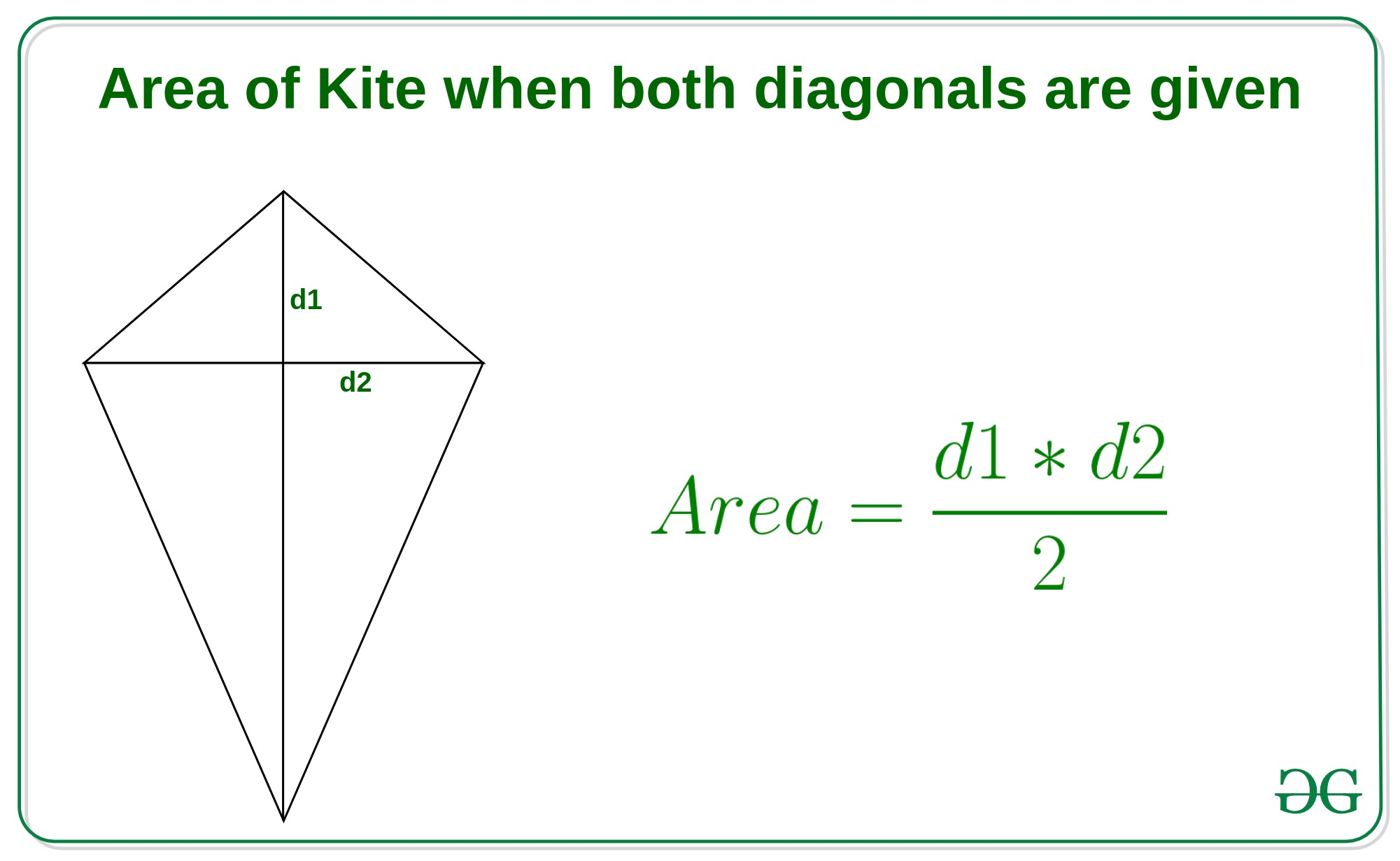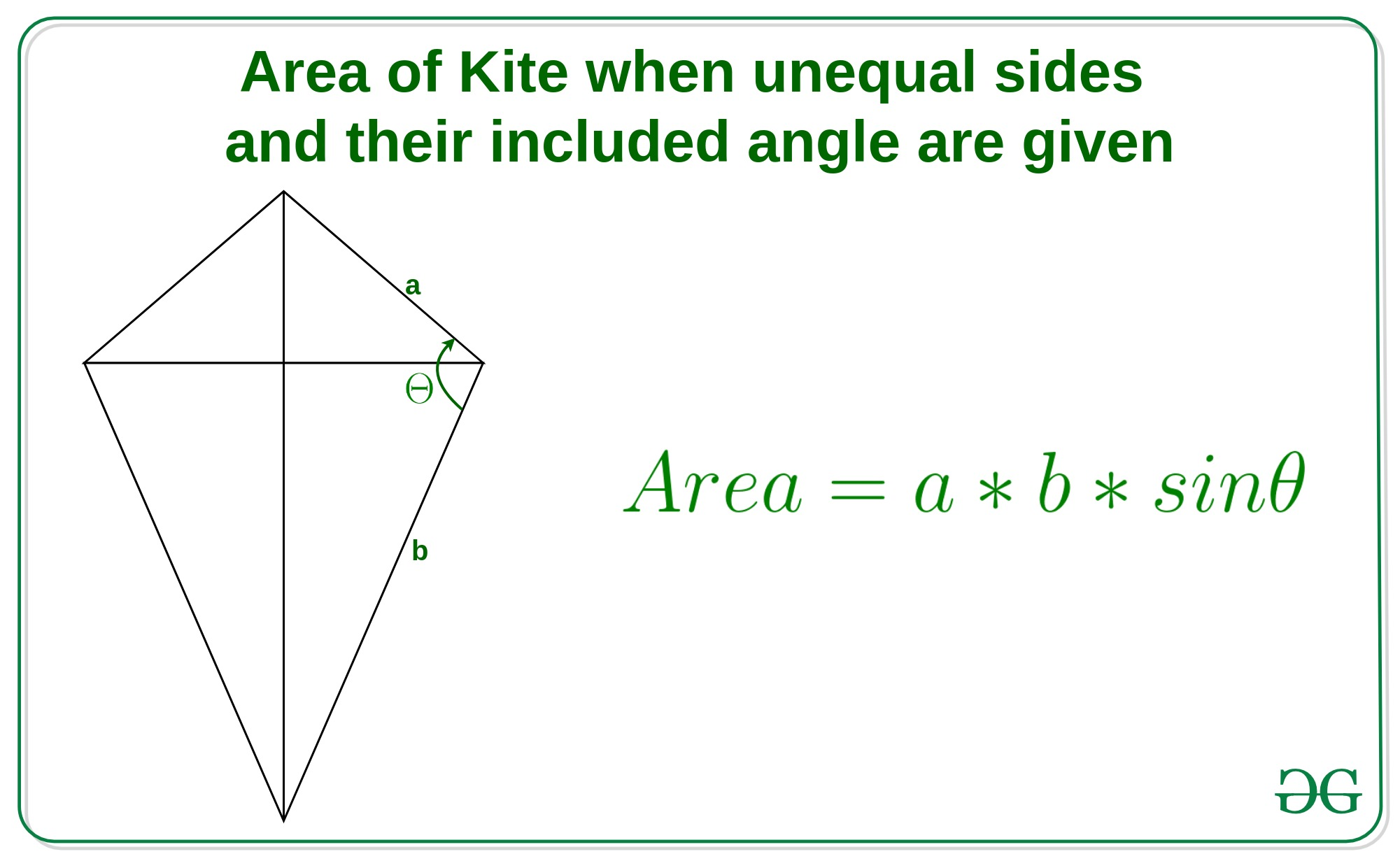# Program to calculate the area of Kite

Kite is something like rhombus but in Kite, the adjacent sides are equal and diagonals are generally not equal.

1. Method 1: When bboth the diagonals are givenIf diagonals d1 and d2 are given of the kite, then the area of a kite is half of product of both the diagonals i.e.Example:

Input: d1 = 4, d2 = 6
Output: Area of Kite  = 12

Input: d1 = 5, d2 = 7
Output: Area of Kite  = 17.5


Approach: In this method we simply use above formula.

Below is the implementation of the above approach:

## C++

 // C++ implementation of the approach     #include  using namespace std;     // Function to return the area of kite  float areaOfKite(int d1, int d2)  {      // use above formula      float area = (d1 * d2) / 2;      return area;  }     // Driver code  int main()  {      int d1 = 4, d2 = 6;      cout << "Area of Kite = "          << areaOfKite(d1, d2);         return 0;  }

## Java

 // Java implementation of the approach  class GFG   {         // Function to return the area of kite      static float areaOfKite(int d1, int d2)      {          // Use above formula          float area = (d1 * d2) / 2;          return area;      }         // Driver code      public static void main(String[] args)      {          int d1 = 4, d2 = 6;          System.out.println("Area of Kite = "                 + areaOfKite(d1, d2));      }  }     // This code is contributed by Rajput-Ji

## Python3

     # Python implementation of the approach     # Function to return the area of kite  def areaOfKite(d1, d2):         # use above formula      area = (d1 * d2) / 2;      return area;     # Driver code  d1 = 4;  d2 = 6;  print("Area of Kite = ",      areaOfKite(d1, d2));     # This code is contributed by Rajput-Ji

## C#

 // C# implementation of the approach  using System;     class GFG   {     // Function to return the area of kite  static float areaOfKite(int d1, int d2)  {      // Use above formula      float area = (d1 * d2) / 2;      return area;  }     // Driver code  public static void Main()  {      int d1 = 4, d2 = 6;      Console.WriteLine("Area of Kite = "             + areaOfKite(d1, d2));  }  }     // This code is contributed by anuj_67..

Output:

Area of Kite = 12

2. Method 2: When side a, b and angle are given:When the unequal sides of kite a and b and the included angle Θ between them are given, thenExample:

Input: a = 4, b = 7, θ = 78
Output: Area of Kite  = 27.3881

Input: a = 6, b = 9, θ = 83
Output: Area of Kite  = 53.5975



Approach: In this method we simply use above formula.

Below is the implementation of the above approach:

## C++

 // C++ implementation of the approach     #include  #define PI 3.14159 / 180  using namespace std;     // Function to return the area of the kite  float areaOfKite(int a, int b, double angle)  {      // convet angle degree to radians      angle = angle * PI;      // use above formula         double area = a * b * sin(angle);      return area;  }     // Driver code  int main()  {      int a = 4, b = 7, angle = 78;      cout << "Area of Kite = "          << areaOfKite(a, b, angle);         return 0;  }

## Java

 // Java implementation of the approach  import java.io.*;     class GFG  {         static double PI = (3.14159 / 180);     // Function to return the area of the kite  static float areaOfKite(int a, int b, double angle)  {      // convet angle degree to radians      angle = angle * PI;             // use above formula      double area = a * b * Math.sin(angle);      return (float)area;  }     // Driver code  public static void main (String[] args)  {         int a = 4, b = 7, angle = 78;      System.out.println ("Area of Kite = " + areaOfKite(a, b, angle));  }  }     // This code is contributed by jit_t.

## Python3

 # Python implementation of the approach  import math  PI = 3.14159 / 180;     # Function to return the area of the kite  def areaOfKite(a, b, angle):             # convet angle degree to radians      angle = angle * PI;             # use above formula         area = a * b * math.sin(angle);      return area;     # Driver code  a = 4; b = 7; angle = 78;  print("Area of Kite = ",          areaOfKite(a, b, angle));     # This code contributed by PrinciRaj1992

## C#

 // C# implementation of the approach  using System;     class GFG  {      static double PI = (3.14159 / 180);     // Function to return the area of the kite  static float areaOfKite(int a, int b, double angle)  {      // convet angle degree to radians      angle = angle * PI;             // use above formula      double area = a * b * Math.Sin(angle);      return (float)area;  }     // Driver code  static public void Main ()  {      int a = 4, b = 7, angle = 78;      Console.WriteLine("Area of Kite = " + areaOfKite(a, b, angle));  }  }     // This code is contributed by ajit

Output:

Area of Kite = 27.3881


Attention reader! Don’t stop learning now. Get hold of all the important DSA concepts with the DSA Self Paced Course at a student-friendly price and become industry ready.

My Personal Notes arrow_drop_upCheck out this Author's contributed articles.

If you like GeeksforGeeks and would like to contribute, you can also write an article using contribute.geeksforgeeks.org or mail your article to contribute@geeksforgeeks.org. See your article appearing on the GeeksforGeeks main page and help other Geeks.

Please Improve this article if you find anything incorrect by clicking on the "Improve Article" button below.